# Project management in costly environments

## Arizona State University

Project managers are brokers in time; because time is money, they constantly trade-off time against penalty costs, bonus (opportunity) costs, changeover costs, expediting as well as de-expediting costs, and more. Today, more than ever before, mistakes appear so costly and rewards for success are so attractive, that almost every decision management undertakes carries with it some real costs of consequence, costs associated with the misapplication of valuable resources over and over again.

A vital issue of modern project management concerns the development of good time estimates for future tasks, good in the sense that on implementation the actual times are in line with previously estimated times. Of course, when they are not, errors arise. Forecasting errors lie at the root of all the important time/cost tradeoffs of scheduling, as managers make moves to compensate for unexpected delays and to capitalize on opportunities as projects unfold.

Good time estimates involve forecasts that compare favourably with actual outcomes and have a reasonable chance of occurring; but beyond this, good forecasts in costly environments must also consider the consequences of being wrong and provide the manager with a reliable bias in the direction of minimizing the costs of forecast errors.

This bias is part of the time/cost trade-off in the real world of project scheduling. There is evidence to show that this bias has already been informally at work in the CPM of the past, but unfortunately it has been sadly lacking in form in PERT procedures. The purpose of this article is to show first how this bias can be easily introduced into PERT, and secondly why it should be part of professional project management today. The procedure has been called BPERT (Bayesian Point Estimation and PERT, see (1) ).

### Costly Environments

Define costly environments as settings in which mistakes are costly! Since mistakes often stem directly from errors of misestimation, forecasts become extremely important. Tasks can be completed both too early and too late. Naturally the costs will be quite different, but nevertheless very real and significant.

Consider an example: a simple task may be defined as, “prepare and complete engineering drawings for part X.” After a careful review of similar tasks in the past, Engineering has suggested ten working days. Again, past experience has shown that there is some doubt that this will happen; the table below shows the historical chances (probabilities) of meeting targets by Engineering.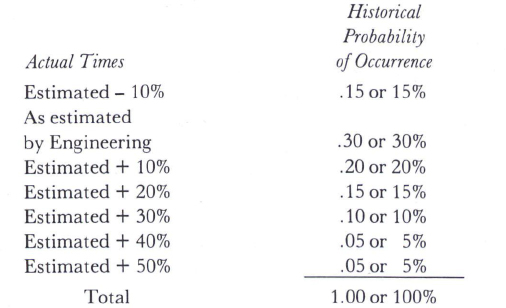Obviously there is a good chance that it will take more than ten working days. Using this past record for planning, the forecast in reality explodes into several potential and meaningful times as show below.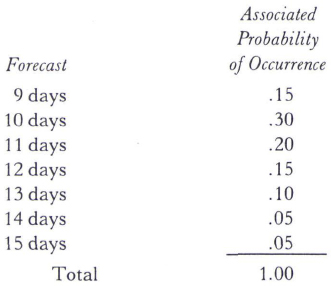Note that the mean forecast (average) is 11.10 days and the median (middle point) is 12 days.

The impact of using the estimated ten days comes into focus when next the costs are considered. Because of the importance of this task and its position in the overall project, costs for actual times later than estimated range around \$200/day. Conversely, actual times that are earlier than estimated times cost only \$100/day. These costs reveal that it is apparently easier to adjust the overall schedules for overestimates (\$100) than for underestimates (\$200).

For this example, the potential costs associated with using the ten day estimate are shown below.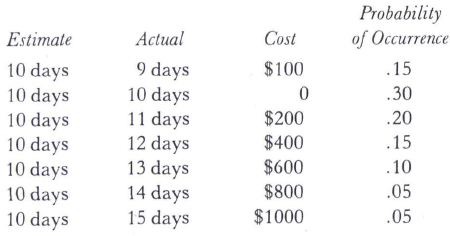There is a real chance that a costly error can occur. Weighting these costs by their probabilities of occurrences, the impact of the expected cost of staying with the ten day estimate is (.15×\$100+ .30 × \$0 + .20 × \$200 + .15 × \$400 + .10 × \$600 + .05 × \$800 + .05 × \$1000) or \$265 for this task alone. Similarly, for any other estimate (for example, mean, median, 13 days, and etc.), a similar impact can be evaluated in expected cost dollars. The approximate mean of 11 days carries an expected cost of \$200; the median of 12 days costs \$195, and so on.

This demonstrates the effects of costly environments. All estimates have associated expected costs relating probabilities to error costs. Once again, let’s define good estimates as those with minimal associated expected costs (of being wrong).

In this case, the mean is not a good estimate. In fact, means usually contribute very little to effective planning in costly environments. This is especially true when the time distributions (probabilities) are skewed as in this example. Averages simply don’t pay-off because they completely disregard cost. Twelve days is a more realistic estimate to plan on, given the situation at hand. Furthermore, if the cost of being late should ever rise to \$2000/ day, an estimate of fourteen or fifteen days would prove to be even better than twelve.

In this sense, good estimates are dependent upon costs as well as probabilities. The differences between 12 days and the mean of 11 days represents safety time, time inserted into the estimate to compensate for the relative costs of \$200/\$100. If the cost ratio rises to \$2000/\$100, more safety time is advised. The concepts at work here are those used to select and size safety stocks in materials planning and control. This idea was first introduced in (1); others in Poland are developing similar approaches to the problem (12). A good treatment of PERT/CPM can be found in the texts (3) and (13).

This next section develops some of the principles of blending costs and probabilities through decision theory.

### Time Estimates for Individual Tasks

All time estimates are future oriented, in that they are made about some planned future task in an unknown environment. Managers must act now:

1. To plan and establish through forecasts (time estimates) target completion dates consistent with reasonable applications of resources (men, materials, and machines) through the technologies at hand.

However, managers must also act at a later date:

2. To control and monitor the evolution of project phases comparing milestones to targeted dates accommodating the expected and the unexpected.

This is where good estimates pay off; the fewer the adjustments the better. It is at this point where PERT has failed as managers report that the three time estimates don’t accommodate these costly adjustments. (See (4), (6), (10), and (11).)

Consider the process of selecting a time estimate for some future activity. The exact time is never known in advance because of the many variables associated with applying resources (men, materials, and machines) to real world tasks. Whenever uncertainty exists, managers try to capture this in probability distributions. The greater the uncertainty, the broader (more diffuse) the distribution. The usual measure of shape is called the standard deviation.

#### PERT

PERT, introduced in the early 1960’s by Kelly (7), is specifically designed to cope with the time uncertainties of task A in figure 1. First the distribution is reduced to a single point estimate, the approximate mean1; in this case it is simply 1/6 (10 + 4 x 20 + 50) or 23.3 days. This is well beyond the most likely (probable) estimate of twenty days because of the influence of a rather high pessimistic view of 54 days.

A standard deviation is often calculated to capture the uncertainty.2 For task A, the standard deviation is 6.67 days.

#### CPM

Again developed in the early 1960’s, CPM approaches the task A in a more pragmatic way. Hopefully through the experience of having completed tasks similar to A in the past, a single point estimate is developed by somehow digesting all of the information in Figure 1.

Other researchers ( (5), (8) and (9) ) have found that that CPM estimates usually are higher (more pessimistic) than PERT estimates. Apparently, CPM estimators immediately see the possibility of an elongated task and, most importantly, see the higher costs of underestimation. Higher estimates build in some safety time. If an error is to occur (and it likely will as experience has shown), it is certainly less costly to estimate on the high side. Corrective action is only \$200/day.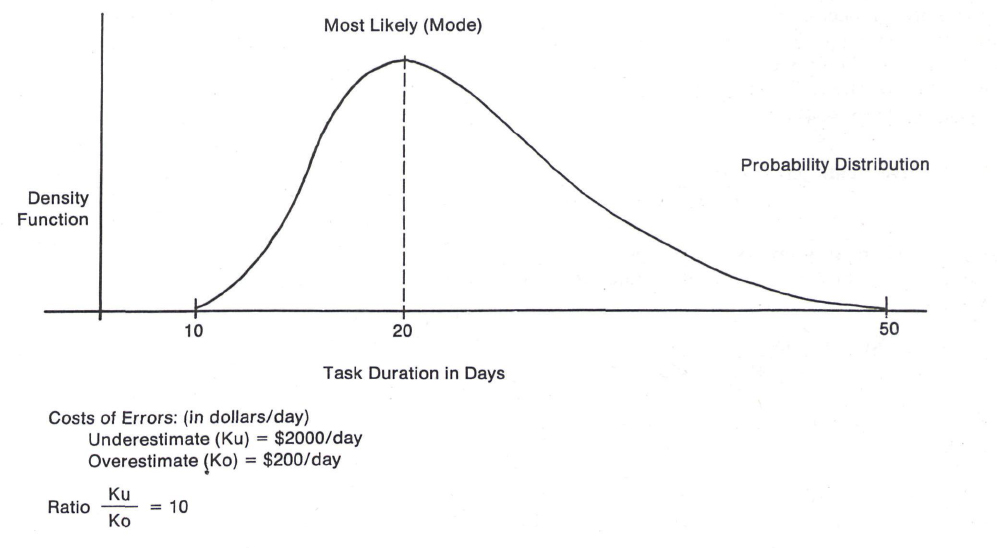Figure 1.

Once again for task A, if 23.3 days is the approximate statistical mean estimate of PERT, then it is not surprising to expect the CPM estimate to be higher. How much higher is the question.

#### Certainty Equivalent

The principles of decision theory suggest that another well-known point estimate is also available, one which minimizes the expected (future) costs of being high or low; this has been called the certainty equivalent (see (14) ). It summarizes both costs and uncertainties into one estimate consistent with good (rational) decision making.3

The certainty equivalent is simply that point which minimizes the expected costs of misestimation. An Appendix summarizes the mathematical derivation of certainty equivalents as optimal point estimates. Fortunately the results are set-up for ease of use in Table I — Loss Ratio and Certainty Equivalents.

Certainty equivalents are obtained as follows. For any task, the uncertainty is first summarized in a standardized mode. This takes into account the location of the most likely estimate in relation to both the optimistic and pessimistic estimates.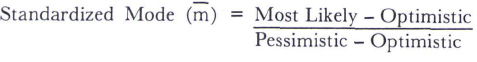Note that this must always lie between zero and one. Next the economics of the situation are summarized in a loss ratio.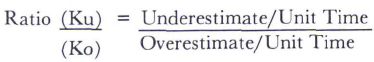Finally, for a given standardized ratio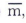search down the column for the appropriate loss ratio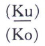and read the certainty equivalent (optimal estimate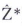) in the left hand column again in standardized form. To complete the calculation: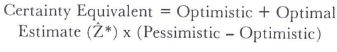Once again for task A,is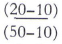or 0.25,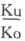and therefore the certainty equivalent is 0.57 in standardized form, and 10 + 0.57 (50-10) or 32.8 days.

The difference between PERT (23.3 days) and the certainty equivalent (32.8 days) shows the amount of safety time required to properly account for the tremendous potential losses of an underestimate. This pessimistic bias of (32.8-23.3) or 10.5 days is the correction factor needed to bring PERT into the real world of dollars and (common) sense. Estimates like 32.8 days are good estimates because they attempt to take into account the environmental risks as reflected in expected costs of misestimation.

Having computed certainty equivalents for each and every task in a project, the project duration can be easily estimated.

### Project Completion Targets

Historically, projects have been viewed as a collection of individual tasks. Project completion dates are estimated through summing the task durations along the critical (longest) path(s). In CPM, the manager gets a single estimate for the project completion date.

However in PERT, the sum of the task mean times along the critical path (s) is purported to give the mean of the project; likewise the sum of variances (standard deviations squared) gives the project variance. Unfortunately, MacCrimmon and Ryavec (10) (as well as many others) have definitely proven this false. The sum of the task mean times and variances are just another set of numbers, and may not even be close to the project mean and variance. This finally crushes the credibility of PERT to provide managers with superior project targets. Probability statements based on the project mean and variance (estimates) may be very misleading.

Apparently in PERT if there is only one critical path that is significantly (several times) longer than any other path, then the estimated project (mean) completion dates are reasonable. However if several paths are competing for the critical path, the estimated project mean will be low. The estimated project variance can be high or low, depending upon the complexity of the network. To date, the only sure way to obtain unbiased project mean and variances is to simulate (15).

The sum of the certainty equivalents for any project along the critical (longest) path(s) represents a target date that has, individually task by task, strived to minimize the expected costs of misestimation. It is a single estimate and provides a good alternative to PERT and CPM.

Table 1 Loss ratios for values of m between .05 and .95 and certainty equivalents z* between .01 and .99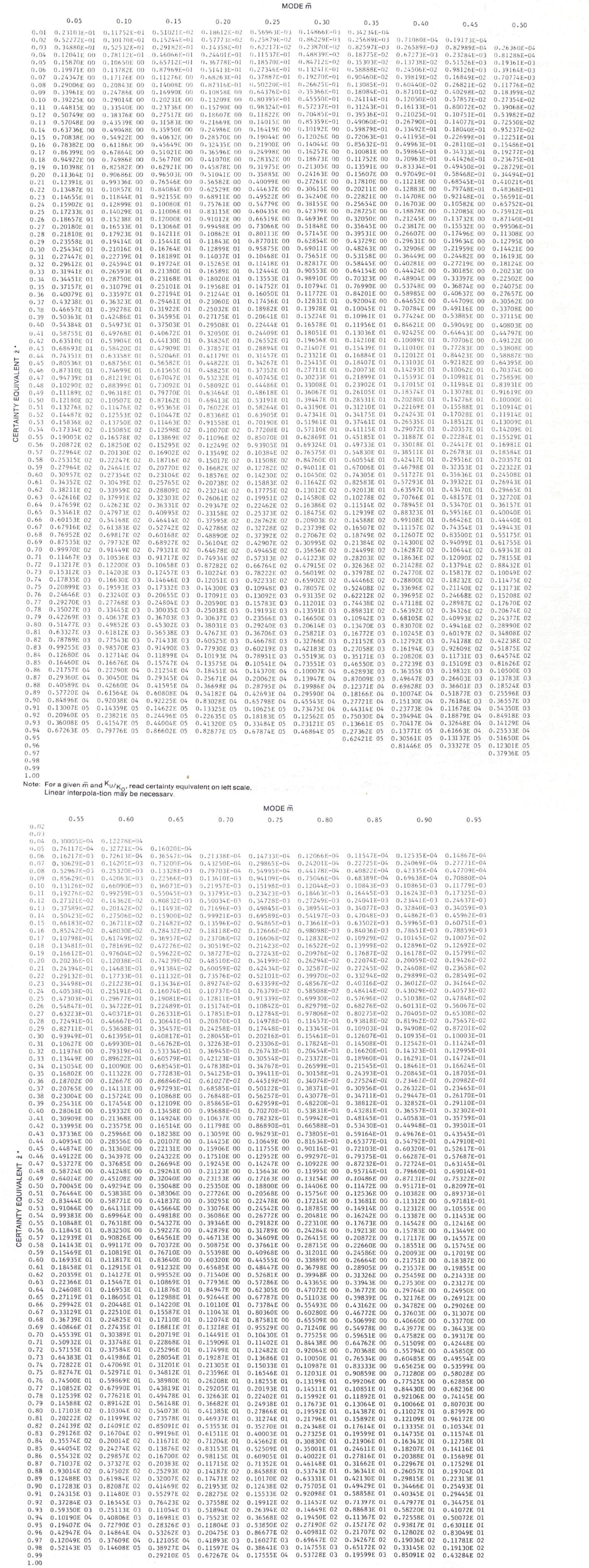A complete discussion and treatment of the project completion dates responding to the certainty equivalents (or Bayesian Point Estimates) is given in (1).

### An Example

In order to visualize the potential impact of certainty equivalents, look at the example of Figure 2. This simple project contains seven tasks, with four paths from start to finish and the usual three time estimates for each activity.

The first important observation is the shape of the times for each task. Activity A is symmetrical, that is the mean, median and mode (most likely) are exactly the same. Activities C, D and E are not symmetrical; they are stretched (skewed) to the right. For each, the mean is larger than the median which in turn is larger than the mode. Finally the remaining activities of B, F and G are each skewed to the left, where for each the mean is less than the median which in turn is less than the mode.

Unfortunately, skewed or stretched distributions are a fact of life. In very few tasks are the optimistic, pessimistic and most likely times symmetrical. Even worse, as activities become skewed the traditional PERT estimates often show significant errors. These are only approximations to the mean. For activity B, the true mean is only 6.45 days whereas the PERT approximated mean is 6.55 days.

When the costs of being wrong are finally considered, the resulting certainty equivalents react to both losses and skewness to give estimates which are different from PERT. A sample calculation of the certainty equivalent is shown below for activity A, where m is the standardized mode (most likely estimate), te the PERT approximation to the mean, and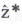,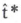the certainty equivalents.

1.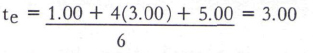2.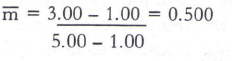3. For Ku/K0 = 2/1 and= 0.500:= .578 using linear interpolation.

4.= 1.00 + .578 (5.00 - 1.00) = 3.312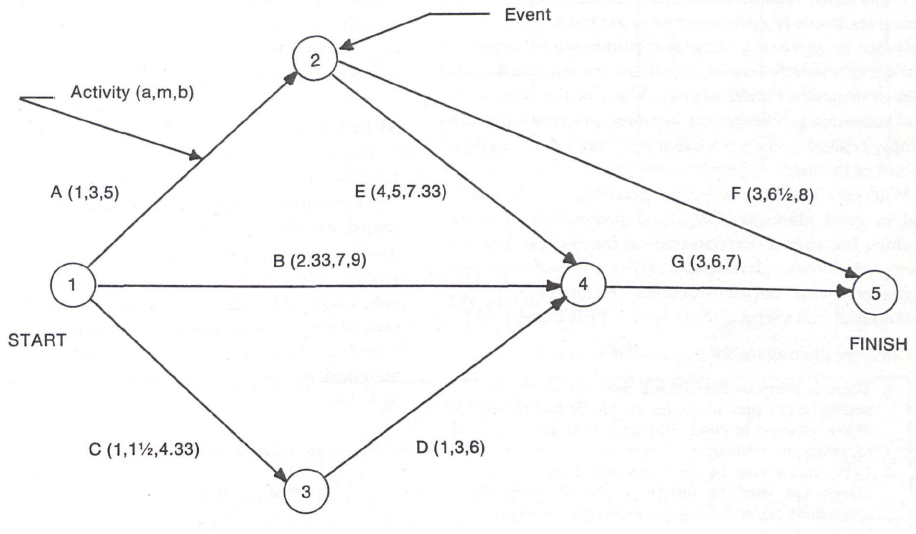Figure 2.
The arrow diagram and three time estimates for the illustrative problem.

Table II summarizes the results for all seven activities of the project. Table III pulls together the result. It is clear that PERT indentifies path A E G with a mean project duration of 13.9 days. The certainty equivalents, on the other hand, suggest path B G with a planning target duration of 14.28 days. The important thing so far is that the critical paths of each procedure are different. These are naturally reflected in float determinations and how they are used to schedule the project.

However, of more importance are the cost implications. If the costs are real, then Table IV summarizes why the certainty equivalents deserve serious attention. The total expected cost of the PERT program is \$32.07, more than twice those of the certainty equivalents. (The formula for the calculations of these losses are given in the Appendix)

Projecting the simple example above into a much larger project of several thousands of activities, it becomes very clear that the introduction of costs will most certainly redirect your attention to other critical paths. These paths will provide target completion dates which can significantly reduce costs of misestimation in project management.

### Conclusions

Project managers must plan ahead and deploy resources in costly environments to get the job done. Not only are there always some uncertainties involved with respect to the tasks ahead, but there are some real costs of misestimation (being wrong). Many of the behavioral and economic problems that surround projects today are simply related to the unfortunate estimates developed on behalf of the user.

Wherever uncertainty exists, probabilities are essential in good planning. Costs and probabilities require nothing less than a decision-making framework. The certainty equivalents, developed earlier, provide project managers with decision-theoretic estimates that are meaningful and useful alternatives to PERT and CPM.

Consider once again the situation to date:

1. There is evidence that PERT does not work; the activity mean time estimates are sterile and made in the absence of costs. Project means and variances are not reliable.

2. CPM appears to be more successful in that it allows the user to informally blend chances (probabilities) and consequences (costs) into one “best” estimate.

3. PERT can be easily upgraded by the addition of a loss structure. The resulting single time estimates, called certainty equivalents, minimize costs of misestimation and develop safety times in response to the cost environment. The process has been called BPERT (Bayesian Point Estimation and PERT(2) ).

4. The certainty equivalents of BPERT can be developed easily from the three time estimates of PERT and a loss ratio input. Tables facilitate the selection of certainty equivalents.

5. Crashing and other issues of PERT/cost can be blended in easily, to yield BPERT/cost.

6. The project duration targets of BPERT are single estimates developed in response to both uncertainties and costs.

Most agree that all project environments have costs of misestimation. The question has been raised, “What if the costs are similar?” If the user is indifferent to the costs of over-and underestimation, then the loss ratio (Ku/Ko) is one and the certainty equivalents become the median, not the mean! In distributions that are not symmetrical, this difference is very real.

In my opinion, this greatly reduces the value of PERT in scheduling. The certainty equivalents of BPERT, in many ways, simply offer a formalized approach to what is probably already being done in good applications of CPM. Instead of simply choosing one estimate based upon years of experience, BPERT clearly lays out the process by which uncertainties and costs combine to give meaningful estimates.

Obviously this approach requires more experiences to be generated and shared along the lines of thinking in terms of probability distributions and identifying more of the real costs of the project management environment.

### APPENDIX:

The following summary of the mathematics of BPERT are taken from (1). For any stochastic project in which the individual activity duration times may be approximated by suitable beta distributions, let the following information is available for each activity: a, b, m and Ku/K0. (Note that it is only necessary to estimate the loss ratio and not the per unit parameters, Ku and K0). Given that the parameters of the beta distribution f(t) are α and β the mode, mean and variance of the revised standardized beta distribution over the interval (0, 1) are as follows: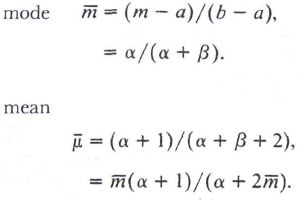Table II.
Resulting statistics of example

 Statistics Activity Ku/Ko m teA 2/1 3.00 3.00 .50 .578 3.31 B 45/1 7.00 6.55 .70 .925 8.50 C 2/1 1.50 1.89 .15 .322 2.07 D 4/1 3.00 3.17 .40 .574 3.87 E 1/1 5.00 5.22 .30 .341 5.14 F 20/1 6.50 6.17 .70 .896 7.48 G 1/1 6.00 5.67 .75 .695 5.78

Table III.
Comparison of critical paths using PERT and certainty equvalents

 Statistics Path Duartion Critical Path Project Duration Est. AF AEG BG CDG PERT te 9.17 13.89 12.22 10.73 AEG 13.89 CERTAINTY EQUIVALENTS10.79 14.23 14.28 11.72 BG 14.28

Table IV.
Comparison of expected costs using PERT and certainty equivalents

 Expected Costs per Activity A B C D E F G Total PERT .94 20.18 .94 2.04 .49 6.29 .49 32.07 CERTAINTY EQUIVALENTS .87 6.68 .80 1.45 .58 3.40 .51 14.29

variance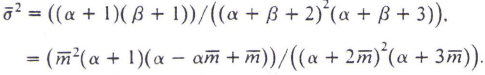The bars over the variable signify that the distribution has been standardized.

An entire family of beta distributions with end points a and b have its mode at m. Their variances will, of course, differ. Finally, assume that the variance of the revised beta distribution σ2 is known. Hence, the parameters of the distribution α and β are obtained from the following equations: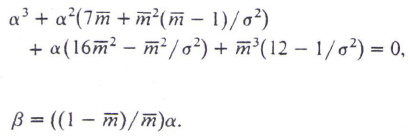The parameters of the loss functions are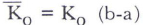and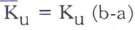. The revised losses are given by: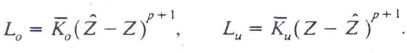Since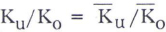, it can be shown that the optimal point estimatefor any standardized unimodal beta function is: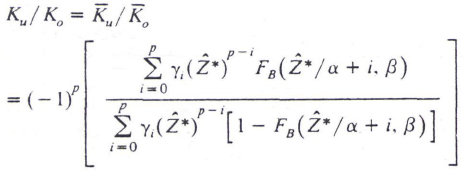where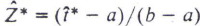optimal point estimate of

standardized beta distribution.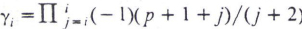and where by definition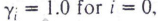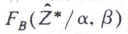=left tail cumulative probability for the standardized

beta distribution, also the “incomplete beta” function

Note that although the power structure is equal for both errors of under and overestimation, p + 1, the per unit parameters of Ku and Ko are not necessarily equal. Hence the loss functions are not mirror images.

In defining point estimates, the shape of the loss structure must be known. In its very simplest form, the loss functions may be linear, p = 0. This is often a reasonable approximation and will be used in this paper for illustrative purposes. Similar formulations to those which follow may be developed for any integer p > 0. Given the added assumptions of the linear loss structure;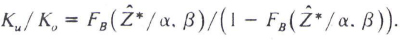When re-written, the optimal estimate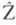* is given a fractile interpretation first introduced in the famous “newsboy” problem: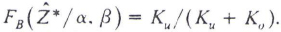One point remains. If the linear loss functions are mirror images, Ku = K0, then the optimal point estimate assumes the median and not the mean. Only under the condition of a symmetrical distribution does the mean also become the optimal point estimate.

For any estimate, the associated expected loss under a linear loss structure using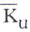and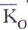is: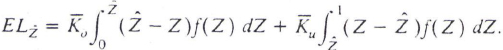By noting that: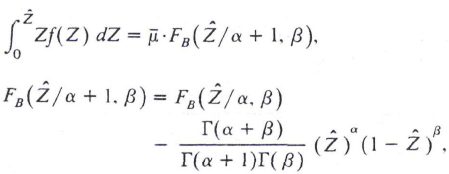where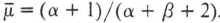the mean of the standardized beta distribution, the expected loss becomes: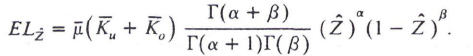The certainty equivalentfor any activity under a linear loss structure and loss ratio Ku/K0 can be readily obtained. Table I relatestofor the linear loss structure case and the PERT-based assumption of an activity standard deviation approximately equal to one-sixth of its range.

The final step in the development of a project duration time is the analysis of path durations E(Pi) and the identification of a critical path through CPM. The project time estimate associated with PBERT becomes E(P1), where: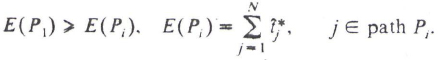E(P1) is a single time estimate for the project, minimizing the sum of the losses in the small for those activities on the critical path.

The application of resources to the various tasks (activities) of a project may take on several levels of intensity. The estimated activity times a, b and m are assuredly functions of the intensity of resource application. In all previous discussions, this dimension of scheduling was left unstirred. Consider briefly the significance of crashing critical activities.

For each activity, define the normal application of resources as the least-cost level of operation. In terms of costs, it represents the most efficient means of performing the activity. Seldom are we interested in the deliberate prolongation of an activity at increased costs; however, of greater interest is the acceleration of activity duration times again at increased costs. Define a crash application of resources as one with costs greater than normal. Several levels of crashing may be feasible culminating in the least-time level of operation without regard to cost.

For each level of resource application, the three estimates a, b and m must be ascertained. As one would expect, under a variety of crashing programs, any and all of a, b and m may change. Similarly, the value of the loss ratio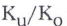may also change significantly. Figure 3 illustrates a situation in which crashing the activity causes the cost of underestimation to increase substantially relative to that of overestimation; the result is a certainty equivalentthat remains relatively unchanged at 4.25 although the PERT activity mean te is reduced by 11%. The reverse may also exist, however, in which the loss ratio and the parameters a, b and m change in a reinforcing manner to cause significant changes in the estimate. Note that explicit considerations must be given to the effects of crashing on the loss ratioas well as a, b and m.

Figure 3.
An illustration of parameter variations in crashing.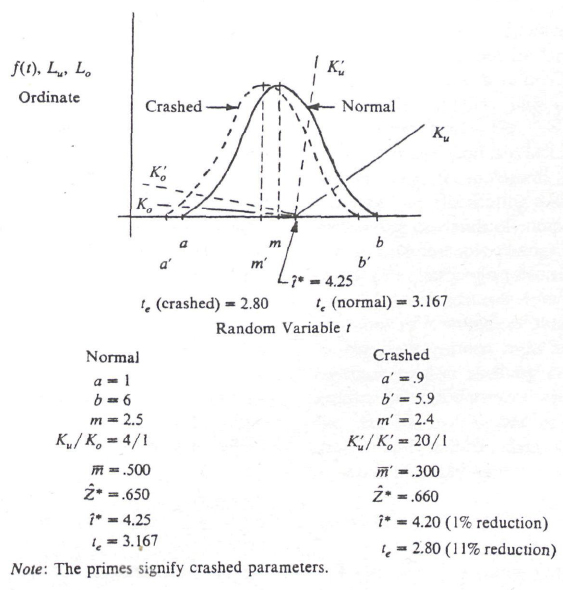### REFERENCES

1. Britney, R. R.. “Bayesian Point Estimation and the PERT Scheduling of Stochastic Activities,” Management Science, 22: 938-948, May 1976.

2. Carruthers, J. A., and A. Battersby, “Advances in Critical Path Methods,” Operational Research Quarterly, 359-380, December, 1966.

3. Chase, R., and Aquilano, N., Production and Operations Management, revised edition, Homewood, Illinois: Richard D. Irwin, Inc., 1977.

4. Davis, E. W., “Project Scheduling Under Resources Constraints — Historical Review,” AIIE Transactions, 5:297-311, December 1973.

5. Ferguson, E. J. and Swift, F. W., “Psychological Advantages of Planning with PERT,” TIMS/ORSA/AIIE Joint National Meetings, November, 1972.

6. Grubbs, F. E., “Attempts to Validate Certain PERT Statistics or ‘Picking on PERT’,” Operations Research, 10:912-915, 1962.

7. Kelly, James E., Jr., “Critical Path Planning and Scheduling: Mathetical Basis,” Operations Research, 9:296-320, May-June, 1961.

8. King, William R., and Talmadge A. Wilson, “Subjective Time Estimates in Critical Path Planning — A Preliminary Analysis,” Management Science, 13:307-320, January, 1967.

9. King, William R., and others, “On the Analysis of Critical Path Time Estimating Behavior,” Management Science, 14:79-84, September, 1967.

10. MacCrimmon, K. R., and C. A. Ryavec, “Analytical Study of the PERT Assumptions,” Operations Research, 12:16-36, January-February, 1964.

11. Neel, C. W., “Evaluation of Network Models Use in Industrial Construction,” IEEE Transactions on Engineering Management, EM 18-18:7-11, February, 1971.

12. Peleck, A., “Risk Optimization on R and D Project Contractual Date Selection,” Conference on Management on Research and Education, Wroclaw, September, 1975.

13. Weist, J. D. and Levy, F. K., A Management Guide to PERT/CPM, Englewood Cliffs, New Jersey: Prentice-Hall, 1969.

14. Winkler, R. L., An Introduction to Bayesian Inferences and Decision, New York: Holt, Rinehart and Winston, Inc., 1972.

15. Van Slyke, R. M., “Monte Carlo Methods and the PERT Problem,” Operations Research, 11:839-860, September 1963.

### ACKNOWLEDGEMENTS

The author wishes to acknowledge the support of the Associate’s Research Fund of the School of Business Administration of the University of Western Ontario as well as the College of Business Administration of Arizona State University.

1Estimate of mean = 1/6 (optimistic + 4 x most likely + pessimistic)=te

2Estimate of standard deviation = 1/6 (pessimistic-optimistic)

3The concept of a rational decision maker is one who always moves to minimize his expected losses in the long run.

## Related Content

• Project Management Journal

### The Dark Side of Environmental Sustainability in ProjectsUsing fraud triangle theory, this study investigated the effects of three types of factors that shape contractor greenwashing behaviors.

• Project Management Journal

### The Dark Side of ProjectsWith this Special Issue and online collection, we aim to open the space for discussion (and more research!) on the dark side of projects and invite you to join our efforts.

• Project Management Journal

### A Qualitative Analysis of Unethical Behaviors in ProjectsThis research critically reviewed project ethics under the philosophical paradigm change from modernism to late modernism.

• Project Management Journal

### The Relationship Between Uncertainty and Task Execution Strategies in Project ManagementCommon project management methodologies do not consider project task uncertainty for determining appropriate task execution strategies.

• Project Management Journal

### The Dark Side of ProjectsThis article presents the dark side of projects, engaging project scholars and practitioners in discussions about sensitive, confusing, uncomfortable, challenging, and questionable phenomena.